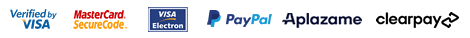▼ Language: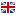English

##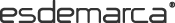WOMAN | MAN | KIDS
Favourites
My account# Buy DIM Valentine's Day for Man

 UP TO 30 FROM 30 TO 60 FROM 60 TO 100 MORE THAN 100
Brands
Sizes

Select
Price

Select
Colour
Select
Style

Select
Material

Select
 Order Relevance Ascending price Descending price Discount New in Delivery date Ratings Sales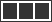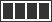### DIM Pack of 2 slips Unno by DIM Basic blue, grey

14.00 €
 Size 3 = 48 4 = 52 5 = 56 6 = 60 Add
Sizes: 3 = 48   4 = 52   5 = 56   6 = 60

### DIM Pack of 2 Unno by DIM Basic boxers blue, grey

14.00 €
 Size 5 = 56 6 = 60 Add
Sizes: 5 = 56   6 = 60

### DIM Pack of 2 Unno by DIM Basic boxers black, fuchsia

14.00 €
 Size 3 = 48 5 = 56 6 = 60 Add
Sizes: 3 = 48   5 = 56   6 = 60

### DIM Pack of 2 Unno by DIM Basic boxers, grey, purple

14.00 €
 Size 6 = 60 Add
Sizes: 6 = 60

### DIM Pack of 2 slips Unno by DIM Basic light blue, marine

14.00 €
 Size 6 = 60 Add
Sizes: 6 = 60

### DIM Pack of 2 black cotton boxers with coloured waistband

23.90 €
 Size 5 = 56 Add
Sizes: 5 = 56

### DIM Pack of 2 Unno by DIM Basic boxers blue, grey

14.00 €
 Size 6 = 60 Add
Sizes: 6 = 60

### DIM Pack of 2 sport ultra-elastic woven boxer shorts green, black

31.90 €
 Size 6 = 60 Add
Sizes: 6 = 60

### DIM Pack of 2 slips Unno by DIM Basic black

14.00 €
 Size 6 = 60 Add
Sizes: 6 = 60

### DIM Pack of 2 slips Unno by DIM Basic white, black

14.00 €
 Size 3 = 48 5 = 56 6 = 60 Add
Sizes: 3 = 48   5 = 56   6 = 60

### DIM Pack of 3 light grey, dark grey, black cotton boxers

35.00 €
 Size 4 = 52 Add
Sizes: 4 = 52

### DIM Pack of 2 Unno by DIM Basic boxers white, black

14.00 €
 Size 4 = 52 5 = 56 6 = 60 Add
Sizes: 4 = 52   5 = 56   6 = 60

### DIM Pack of 2 slips Unno by DIM Basic blue, black

14.00 €
 Size 3 = 48 4 = 52 6 = 60 Add
Sizes: 3 = 48   4 = 52   6 = 60

### DIM Pack of 2 slips Unno by DIM Basic fuchsia, black

14.00 €
 Size 3 = 48 4 = 52 6 = 60 Add
Sizes: 3 = 48   4 = 52   6 = 60

### DIM Pack of 2 cotton boxer shorts with striking blue print waistbands

20.90 €
 Size 3 = 48 4 = 52 Add
Sizes: 3 = 48   4 = 52

### DIM Pack of 2 long sport boxer shorts, ultra elastic fabric, white, grey

31.90 €
 Size 2 = 44 3 = 48 4 = 52 Add
Sizes: 2 = 44   3 = 48   4 = 52

### DIM Pack of 2 Unno by DIM Basic marine boxers, black

14.00 €
 Size 6 = 60 Add
Sizes: 6 = 60

### DIM Pack of 2 Unno by DIM Basic boxers blue, grey

14.00 €
 Size 3 = 48 5 = 56 6 = 60 Add
Sizes: 3 = 48   5 = 56   6 = 60

### DIM Pack of 3 black elastic cotton slips

26.90 €
 Size 2 = 44 3 = 48 4 = 52 5 = 56 6 = 60 Add
Sizes: 2 = 44   3 = 48   4 = 52   5 = 56   6 = 60

### DIM Pack of 2 black elastic cotton boxers

31.90 €
 Size 3 = 48 4 = 52 5 = 56 6 = 60 Add
Sizes: 3 = 48   4 = 52   5 = 56   6 = 60

### DIM 2-pack of blue, grey motion-adjustable boxer shorts

31.90 €
 Size 2 = 44 3 = 48 4 = 52 5 = 56 6 = 60 Add
Sizes: 2 = 44   3 = 48   4 = 52   5 = 56   6 = 60

### DIM Pack of 2 sport boxers, ultra elastic fabric, white, grey

31.90 €
 Size 2 = 44 3 = 48 4 = 52 5 = 56 Add
Sizes: 2 = 44   3 = 48   4 = 52   5 = 56

### DIM Pack of 2 slips Unno by DIM Basic marine, grey

14.00 €
 Size 6 = 60 Add
Sizes: 6 = 60

### DIM Pack of 2 slips Unno by DIM Basic burgundy, black

14.00 €
 Size 5 = 56 6 = 60 Add
Sizes: 5 = 56   6 = 60

### DIM Pack of 2 cotton boxer shorts with striking grey printed waistbands

20.90 €
 Size 3 = 48 4 = 52 5 = 56 Add
Sizes: 3 = 48   4 = 52   5 = 56

### DIM Pack of 2 long sport boxers, ultra elastic marine fabric, red

31.90 €
 Size 2 = 44 3 = 48 4 = 52 5 = 56 Add
Sizes: 2 = 44   3 = 48   4 = 52   5 = 56

### DIM Pack of 2 Unno by DIM Basic boxers, light blue, marine

14.00 €
 Size 6 = 60 Add
Sizes: 6 = 60

### DIM Pack of 2 Unno by DIM Basic boxers, burgundy, black

14.00 €
 Size 5 = 56 6 = 60 Add
Sizes: 5 = 56   6 = 60

### DIM Pack of 3 gray, red, black elastic cotton slips

26.90 €
 Size 3 = 48 4 = 52 5 = 56 6 = 60 Add
Sizes: 3 = 48   4 = 52   5 = 56   6 = 60

### DIM Pack of 2 light blue elastic cotton boxers

31.90 €
 Size 5 = 56 Add
Sizes: 5 = 56

### DIM Pack of 3 light blue, navy, blue cotton boxers

35.00 €
 Size 3 = 48 6 = 60 Add
Sizes: 3 = 48   6 = 60

### DIM Pack of 2 Unno by DIM Basic black boxers

14.00 €
 Size 2 = 44 6 = 60 Add
Sizes: 2 = 44   6 = 60

### DIM Pack of 2 slips Unno by DIM Basic black

14.00 €
 Size 3 = 48 4 = 52 5 = 56 6 = 60 Add
Sizes: 3 = 48   4 = 52   5 = 56   6 = 60

### DIM Pack of 2 long sports microfibre boxers with breathable mesh grey, black

29.90 €
 Size 2 3 4 Add
Sizes: 2   3   4

### DIM Pack of 2 black elastic cotton boxers

35.00 €
 Size 3 = 48 Add
Sizes: 3 = 48

### DIM Pack of 3 red, blue, navy elastic cotton boxers

39.90 €
 Size 2 = 44 4 = 52 Add
Sizes: 2 = 44   4 = 52

### DIM Pack of 2 blue combed cotton boxers, navy

31.90 €
 Size 2 = 44 3 = 48 4 = 52 5 = 56 6 = 60 Add
Sizes: 2 = 44   3 = 48   4 = 52   5 = 56   6 = 60

### DIM Pack of 2 blue seamless slips

25.90 €
 Size 3 = 48 Add
Sizes: 3 = 48

### DIM Pack of 3 black, grey, white elastic cotton slips

26.90 €
 Size 2 = 44 3 = 48 4 = 52 5 = 56 6 = 60 Add
Sizes: 2 = 44   3 = 48   4 = 52   5 = 56   6 = 60

### DIM Pack of 2 marine ultra-stretch sport boxer shorts, red

31.90 €
 Size 2 = 44 4 = 52 6 = 60 Add
Sizes: 2 = 44   4 = 52   6 = 60

 Order Relevance Ascending price Descending price Discount New in Delivery date Ratings Sales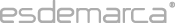Esdemarca Esdemarca Our commitments Privacy policy About cookies Your orders Help Security Shipping costs Easy return Contacts Are you a supplier? Join our team Customer service Make your suggestion Connect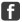Facebook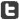Twitter Go to mobile versionEsdemarca · Copyright © Fusion Lab, S.L. 2021  |  Legal warning B66138827 · Calle Manzanares, 4, 28005, Madrid (España) · consultas@esdemarca.com · +34 91 769 72 17